### Home > INT2 > Chapter 12 > Lesson 12.2.3 > Problem12-114

12-114.
1. For each of the relationships represented in the diagrams below, write and solve an equation for x and/or y. Justify your method. In part (a), assume that C is the center of the circle. Homework Help ✎

2.  a.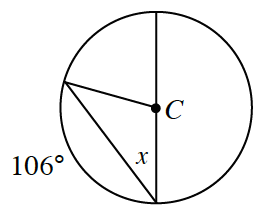b.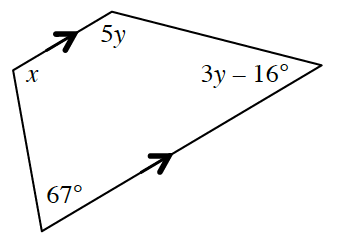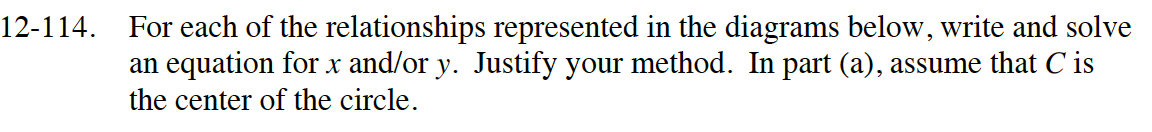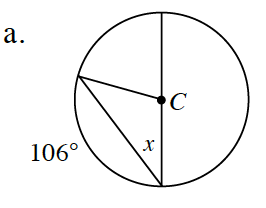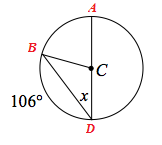$\widehat{ABD} = 180^\circ (\text{because\:} AD \text{\:is\:a\:diameter)}$

$\widehat{AB} + \widehat{BD} = \widehat{ABD}$

$106^\circ + BD = 180^\circ$

$BD = 74^\circ$

$\text{The\:intercepted\:arc\:is\:twice\:the\:inscribed\:angle}$

BD = 2(x)
74 = 2x
x = 37°

$\text{One\:equation\:could\:be\:} 2x = 180^\circ - 106^\circ$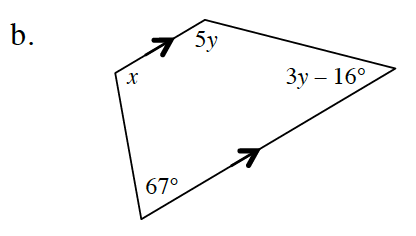Which angles are supplementary? This will help you write equations to solve for x and y.